# Central Limit Theorem

### This theorem fundamental in statistics. It says that the sum of a sufficiently large number of independent and identically distributed random variables follows a normal distribution.

· Big Data Zone · Analysis
Save
5.47K Views

The Encyclopedia of Educational Research, Measurement, and Evaluation (edited by Bruce B. Frey) is out...Bruce kindly asked me to write an entry on the central limit theorem in the encyclopedia...

The central limit theorem is a fundamental theorem of statistics. It prescribes that the sum of a sufficiently large number of independent and identically distributed random variables approximately follows a normal distribution.

## History of the Central Limit Theorem

The term "central limit theorem" most likely traces back to Georg Pólya. As he recapitulated at the beginning of a paper published in 1920, it was "generally known that the appearance of the Gaussian probability density exp(-x^2) in a great many situations" can be explained by one and the same limit theorem, which plays "a central role in probability theory." Laplace had discovered the essentials of this fundamental theorem in 1810 and with the designation "central limit theorem of probability theory," which was even emphasized in the paper's title. Pólya gave it the name that has been in general use ever since.

In this paper of 1820, Laplace starts by proving the central limit theorem for some certain probability distributions. He then continues with arbitrary discrete and continuous distributions. But a more general (and rigorous) proof should be attributed to Siméon Denis Poisson. He also intuited that a weaker version could easily be derived. As for Laplace, the main purpose of that central limit theorem for Poisson was to be a tool in calculations, not so much to be a mathematical theorem in itself. Therefore, neither Laplace nor Poisson explicitly formulates any conditions for the theorem to hold. The mathematical formulation of the theorem is due to the St. Petersburg School of probability, from 1870 until 1910, with Chebyshev, Markov, and Liapounov.

## Mathematical Formulation

Let: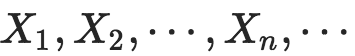...be independent random variables that are identically distributed, with mean μ and finite variance σ2. Let: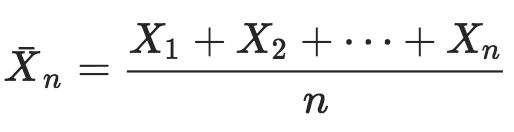...then from the law of large numbers: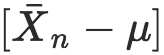...tends to 0 as n tends to infinity. The central limit theorem establishes that the distribution of: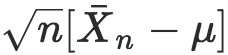...to a centered normal distribution when n goes to infinity. More specifically: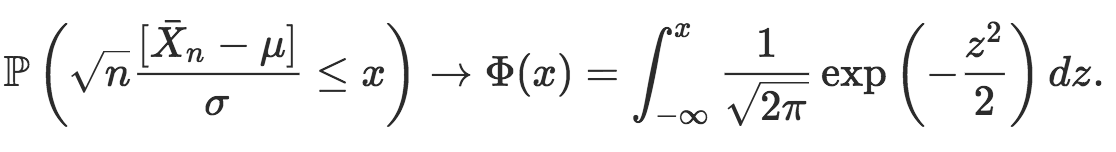We can also write: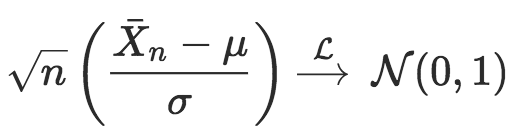Or: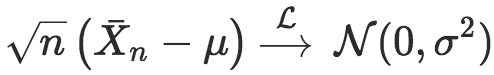## A Limiting Result as an Approximation

This central limit thereom is used to approximate distributions derived from summing, or averaging, identical random variables.

Consider, for instance, a course where seven students out of eight pass. What is the probability that (at least) four failed in a class of 25 students. Let X be the dichotomous variable that describes failure: 1 if the student failed and 0 if they passed. That random variable has a Bernoulli distribution with parameter p=1/8, with mean 1/8, and variance 7/64. Consequently, if students' grades are independent, then the sum: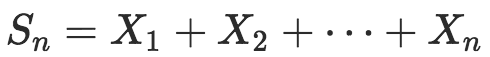...follows a binomial distribution, with mean np and variance np(1-p), which can be approximated by the central limit theorem, by a normal distribution with mean np and variance np(1-p). Here, μ=3.125  while σ^2=2.734. To compute: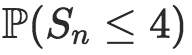...either enter the binomial distribution or the Gaussian approximation. In the first case, the probability is 80.47%.

In the second case, use a continuity correction, and compute the probability that Sn is less than 4+1/2. From the central limit theorem: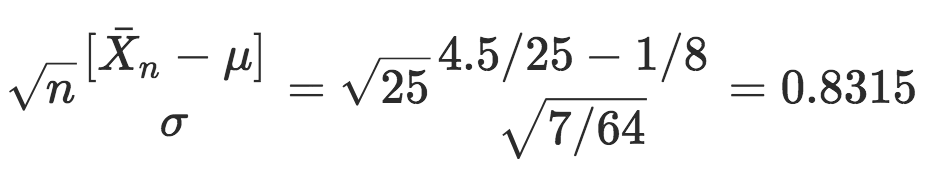The probability that a standard Gaussian variable is less than this quantity is: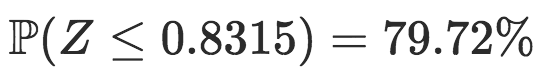...which can be compared with 80.47% obtained without the approximation, see Figure 1. Note that this approximation was obtained by De Moivre in 1713 and is usually known as Bernoulli's law of large numbers.Figure 1: Gaussian approximation of the binomial distribution.

## Asymptotic Confidence Intervals

The intuition is that a confidence interval is an interval in which one may be confident that a parameterof interest lies. For instance, that some quantity is measured , but the measurement is subject to a normally distributed error, with known variance σ2. If X has a: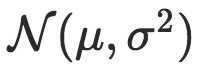...distribution, we know that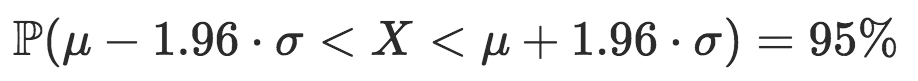Equivalently, we could write: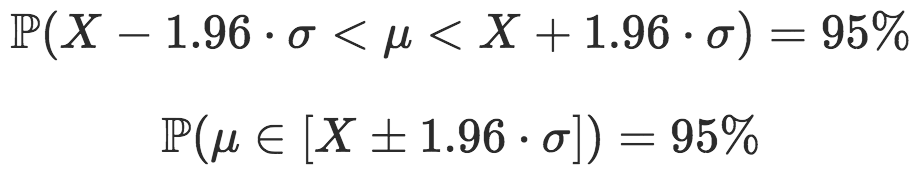Thus, if X is measured to be x, then the 95% confidence interval for μ is: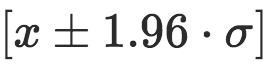In the context of Bernoulli trials (described above), the asymptotic 95% confidence interval for p is: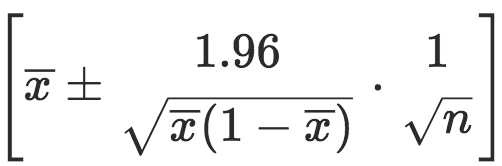A popular rule of thumb can be derived when p~50%. In that context: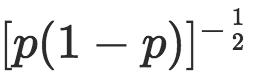...is close to 1.96 (or 2), and a 95 % approximated confidence interval is then: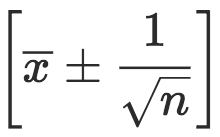See Figure 2. If that confidence interval provides a good approximation for the 95% confidence interval when p~50 %, it is an over-estimation when p is either much smaller or much larger.Figure 2: Law of large numbers on the left, with the convergence of: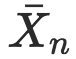...towards p as n increases, and central limit theorem, on the right, with the convergence of: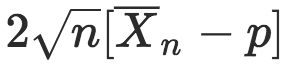...towards a Gaussian distribution. The red area is the 95% confidence region.

## The Delta Method and Method of Moments

This method is used to approximate a general transformation of a parameter that is known to be asymptotically normal: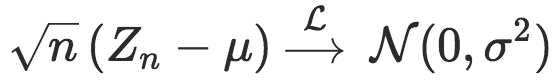...then: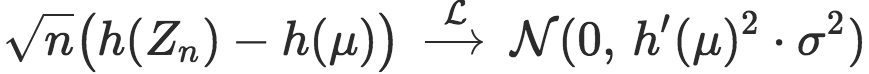Consider now a parametric model, ... independent, with identical distribution: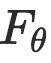(which can be a Weibull distribution to model a duration, a Pareto distribution to model the income or the wealth, etc.). The method of moments is a method of estimating parameters based on equating population and sample values of certain moments of the distribution. For instance, if: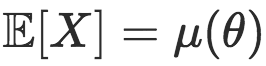...then the estimator: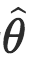...of the unknown parameter is given by the equation: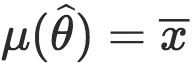...or equivalently: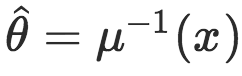From the central limit theorem: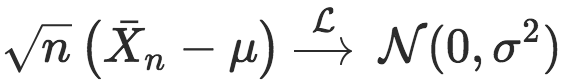...and applying the delta-method with
h=\mu^{-1}
h=μ^1, then: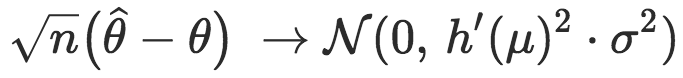...where a numerical approximation for the variance can be derived. This method has a long history and has been intensively studied. Furthermore, this asymptotic normality can be used to compute a confidence interval and also to derive an asymptotic testing procedure.

## An Asymptotic Testing Procedure

Based on that asymptotic normality, it is possible to derive a simple testing procedure. Consider a test of the hypothesis: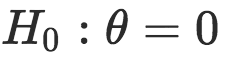...against:...usually called a "significant" test for parameter θ (or significance of an explanatory variance in the context of regression model). Under the assumption that: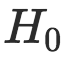...is valid, then: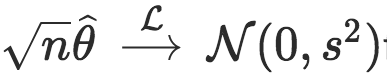...for some variance s^2, that can be computed using the delta method. The p-value associated with that test is: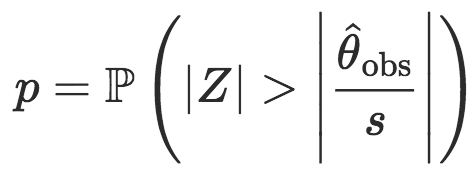...where: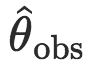...is the observed empirical estimator of the parameter and Z is a standard normal variable. Thus, the p-value can easily be computed using quantiles of the standard normal distribution. Here, the p-value is above 5% if: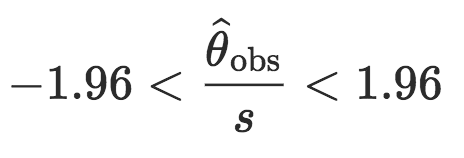## Weaker Forms of the Central Limit

As stated by Laplace, the Central Limit Theorem relies on strong assumptions. Hopefully, most of them can be relaxed. In a first variant of the theorem, random variables have to be independent, but not necessarily identically distributed. If random variables: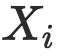...have averages: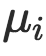...and: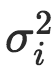...then μ and σ^2 in the Central Limit Theorem should be replaced by averages of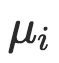...and: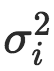...with an additional technical assumption related to the existence of some higher moments (the so-called Lyapounov condition).

For a second variant of the theorem, random variables can be dependent, as in ergodic Markov chain, or in autoregressive time series. In that context, if:...is a stationary time series with mean \mu, then define: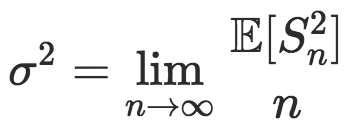...and with that limit, the central limit theorem holds: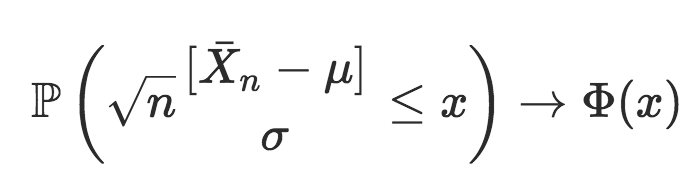...even if the variance term has here a different interpretation.

Finally, a third variant that can be mentioned is the one obtained by Paul Lévy about asymptotic properties of the empirical average when the variance is not finite (actually, even when the first moment is not finite). In that case, the limiting distribution is no longer Gaussian.

Topics:
big data, statistics, central limit theorem

Published at DZone with permission of Arthur Charpentier, DZone MVB.

Opinions expressed by DZone contributors are their own.